3.28.2016

Degenerate triangle

Finally, we will check the law of cosines to the perimeter in the degenerate triangle. There can be two options. If we decrease the base of an isosceles triangle to zero, we obtain the two overlapping segments. The sum of the angles of this degenerate triangle is 180 degrees.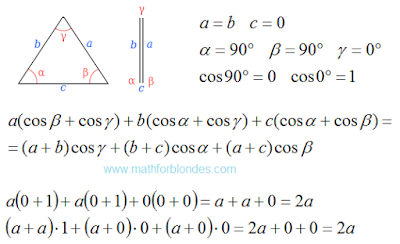Degenerate triangle

If we combine the upper vertex of the triangle with the base, we get the second type of degenerate triangles. This segment equal to the sum of the other two segments. The sum of the angles are also equal to 180 degrees.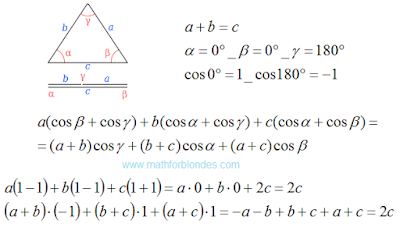Degenerate triangle

A degenerate triangle - is the lower limit of the application of the law of cosines. After that we will look at the law of cosines in general.

3.24.2016

Isosceles obtuse triangle

Now we will check how the law of cosines in obtuse triangle. For example, consider an isosceles obtuse triangle.Isosceles obtuse triangle

Ups! The perimeter of the triangle may be square roots. The law of cosines is not working? Do not jump to conclusions. The secret is revealed very simple. Let us express the base of the triangle through the sides and see what happens. Double-paste the resulting equation in our result. First time turvy-topsy, second time topsy-turvy.The perimeter of the triangle

The law of cosines to the perimeter works flawlessly. We have completed our review of the degenerate triangle.

P.S. 05/31/16 The онлайн игрыcalculator of triangles confirms the received result.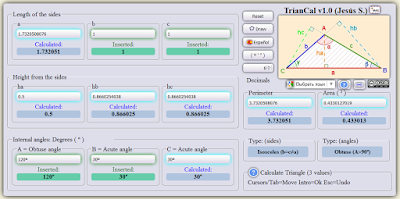To calculate a triangle

3.16.2016

Right triangle

Now we will check the law of cosines to the perimeter of the example of a right triangle. At one corner of the right triangle is 90 degrees, the cosine of this angle is zero. Cosine of the other angles are obtained by dividing the length of the hypotenuse to the length of the adjacent side. In general, the law of cosines check looks like.Right triangle

Now we substitute in formula for the length of the sides of a right triangle and values of cosines.Right triangle

These values are equal to the perimeter of the triangle. Cosine law allows to check the triangle on the break. That's what happens when one of the sides does not reach the top of the triangle.Broken triangle

Equality is performed, but the result is not equal to the sum of the lengths of a broken line or the perimeter of the triangle. You can then proceed to test the law of cosines in the isosceles obtuse triangle.

3.15.2016

Check the law of cosines

Check the law of cosines for the perimeter, we start with an equilateral triangle. All sides of an equilateral triangle are equal. All angles are equal to 60 degrees. The perimeter of the triangle is equal to three times the length of the sides.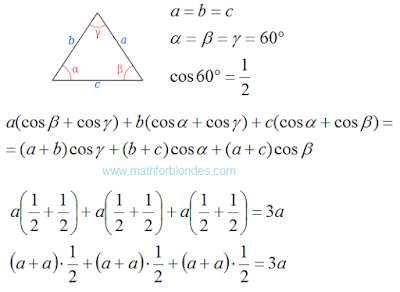Equilateral triangle

Equality is performed. Now we check the law of cosines in a right triangle.

3.14.2016

Law of cosines for perimeter

Carefully look at the proof of the law of cosines and make some corrections.Analysis of proof

Now we can write the law of cosines for the perimeter of the triangle.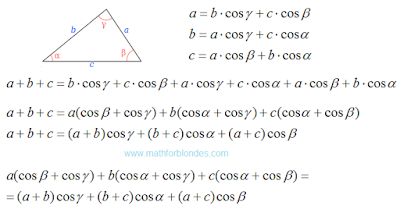Law of cosines for perimeter

The result is a very simple and beautiful formula that describes the entire triangle. The law of cosines shows a relationship between the angles and the lengths of the sides of a triangle.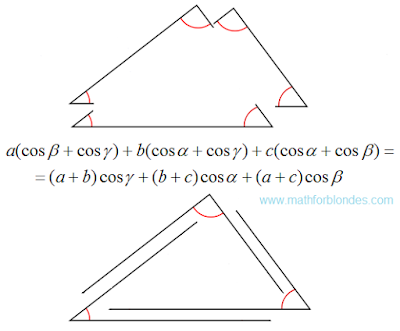The geometry of the law of cosines

Law of cosines for perimeter describes the perimeter of the triangle made up of one-dimensional Euclidean spaces. For multidimensional spaces cosine law has a different view.

3.13.2016

Law of cosines

The triangle has three sides and three corners. Appearance cosine theorem depends on the received angles and sides of the triangle symbols. Here's how it looks in Wikipedia.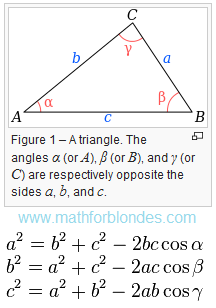Law of cosines

Three angle of the triangle gives three options for the formula of one triangle. In law of cosines can use a one formula, and three variants of symbols.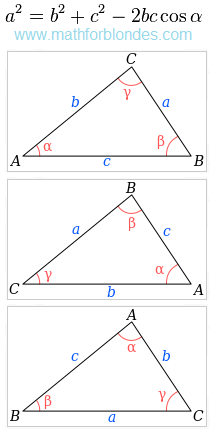Three variants of symbols

These two options allow to describe all sides and angles of the triangle. The traditional problems of mathematics we are taught to find one of the triangle elements.

Question: Can one formula with one variant of symbols to describe all the elements of the triangle?

Here's how to do it using the cosine theorem.

The proof of the theorem of cosines in the trigonometric form looks like.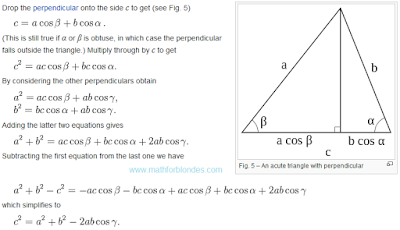The proof using trigonometry

If you change the "minus" sign in the "plus" sign, we get the cosine theorem for the perimeter of the triangle.

Law of cosines in general form (in Russian).

3.04.2016

What are the angles of the triangle?

Q: What are the angles of the triangle ABC, if the sum of the angles A and B is equal to 100 degrees, the sum of the angles B and C equal to 120 degrees?The triangle and the angles
We have two sum of two angles:

A + B = 100
B + C = 120

The sum of angles in a triangle is 180 degrees.

A + B + C = 180

Substitute in this formula the sum of two angles and find the third angle:

100 + C = 180
C = 180 - 100
C = 80

The second sum of the angles substitute angle C:

B + 80 = 120
B = 120 - 80
B = 40

The first sum of angles substitute angle B:

A + 40 = 100
A = 100 - 40
A = 60

A: The angles of the triangle are equal: A=60 degrees, B=40 degrees, C=80 degrees.

If we substitute in the second sum angles of a triangle, then the solution would be:

A + 120 = 180
A = 180 - 120
A = 60

60 + B = 100
B = 100 - 60
B = 40

60 + 40 + C = 180
C = 180 - 60 - 40
C = 80

The third variant of the decision:

We find the angle A of the first sum.

A + B = 100
A = 100 - B

We find the angle C of the second sum.

B + C = 120
C = 120 - B

Substitute the found angles to the general formula:

A + B + C = 180
(100 - B) + B + (120 - B) = 180
100 - B + B + 120 - B = 180
B - 2B = 180 - 100 - 120
-B = -40
B = 40

A = 100 - 40
A = 60

C = 120 - 40
C = 80

Conclusion: if the problem is solved correctly, the result does not depend on the method of solution.

What time is it now?

Q: From the beginning of the day it took 3/4 days. What time is it now?

The day has 24 hours. Multiply the fraction by the number of hours in the day.

24 * 3/4 = 18 (hours)

From the beginning of the day it took 18 hours. Now we can calculate the time.

18:00 - 12:00 am = 6:00 pm

A: Now 18:00 or 6:00 pm.

3.01.2016

How to simplify?

Help simplify the mathematical expressions:

1) 5.25x+7.56+4.98x-1.25x+0.02x
2) 12.78x+3.84x+2.22x+1.16x-0.3x

It is not hard. We believe that X's - it's rabbits. Why rabbits are not the whole? Their evil Putin nibbled. The numbers - it's just a numbers. Now we add up the rabbits with rabbits. The numbers add up separately. Perform a permutation terms, make it easier to summarize.

1) 5.25x+7.56+4.98x-1.25x+0.02x=
=(5.25x-1.25x)+(4.98x+0.02x)+7.56=
=4x+5x+7.56=9x+7.56

2) 12.78x+3.84x+2.22x+1.16x-0.3x=
=(12.78x+2.22x)+(3.84x+1.16x)-0.3x=
=15x+5x-0.3x=19.7x

Here's how it's simple.